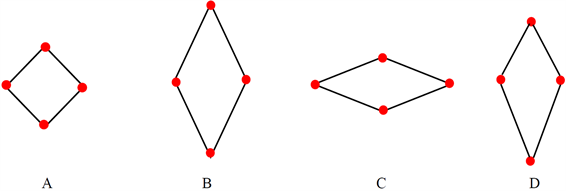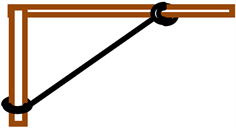﻿ 非惯性系在高中力学解题的妙用

# 非惯性系在高中力学解题的妙用The Application of Non-Inertial System in Solving Mechanics Problems in Senior High School

Abstract: Training of thinking ability in physics teaching is an important way to improve students’ scientific literacy in middle school. Based on the perspective of exploring teaching strategies of high school physics methods, this paper analyzes and compares the solutions of four typical mechanical problems in senior high school with those of inertial system and non-inertial system respectively. It is expected to be helpful to the improvement of physics teaching methods and the improvement of students’ thinking ability.

1. 引言

2. 惯性系与非惯性系

3. 惯性系在高中力学题目解题的局限性

4. 选用惯性系与非惯性系的解题对比

4.1. 抛体问题Figure 1. Title example

4.2. 追及相遇问题

A. ${a}_{1}={a}_{2}$，A、B相遇一次。 B. ${a}_{1}>{a}_{2}$，A、B相遇两次。

C. ${a}_{1}<{a}_{2}$，A、B可能不会相遇。 D. ${a}_{1}<{a}_{2}$，A、B可能相遇一次。

${a}_{1}\ne {a}_{2}$ 时，为关于时间的一元二次方程。由求根公式得 $t=-\frac{2{v}_{0}±\sqrt{4{v}_{0}^{2}+8\left({a}_{1}-{a}_{2}\right)L}}{2\left({a}_{1}-{a}_{2}\right)}$。当 ${a}_{1}>{a}_{2}$，只有一个正根，即两车相遇一次；当 ${a}_{1}<{a}_{2}$，若 $\Delta <0$，方程无解，两车不相遇，若 $\Delta =0$，只有一个正根，即两车相遇一次，若 $\Delta >0$，方程有两解，可能有一个正根，可能有两个正根，因此可能相遇一次，可能相遇两次。

4.3. 滑块木板问题Figure 2. Title example

A先向左做匀减速直线运动至速度为零，后相对于地面向右做匀加速直线运动，加速度大小仍为 ${a}_{A}=4\text{\hspace{0.17em}}\text{m}/{\text{s}}^{\text{2}}$。B一直向右做匀减速直线运动。当A相对地面速度为零经历时间 ${t}_{1}=0.5\text{\hspace{0.17em}}\text{s}$，此时 ${v}_{B}=1.5\text{\hspace{0.17em}}\text{m}/\text{s}$。A、B再次共速经历时间为 ${t}_{2}$，代入公式可解得 ${t}_{2}=0.3\text{\hspace{0.17em}}\text{s}$。从零时刻到A、B共速过程利用位移公式可以得 $L={S}_{A}+{S}_{B}=0.32\text{\hspace{0.17em}}\text{m}+1.28\text{\hspace{0.17em}}\text{m}=1.60\text{\hspace{0.17em}}\text{m}$

A相对于B的初速度 ${v}_{AB}=4\text{\hspace{0.17em}}\text{m}/\text{s}$ 方向水平向左，A相对于B的加速度 ${a}_{AB}=5\text{\hspace{0.17em}}\text{m}/\text{s}$。当A、B共速时，A相对于B的速度为零。则利用速度位移公式得 $L=1.60\text{\hspace{0.17em}}\text{m}$

4.4. 牵连运动问题Figure 3. Title example

${a}_{AB}={a}_{B}\mathrm{cos}\theta -{a}_{A}\mathrm{sin}\theta$。根据 ${v}_{AB}^{2}={a}_{AB}l$，可得 ${a}_{B}={a}_{A}\mathrm{tan}\theta +\frac{{v}_{A}^{2}}{l{\mathrm{cos}}^{3}\theta }$

5. 结语

 程守洙, 江之永. 普通物理学 [M]. 第6版. 北京: 高等教育出版社, 2016: 42.

 刘安巍, 付强. 利用非惯性参考系求解高中物理典型习题初探[J]. 林区教学, 2018(1): 67-69.

 王永柱. 力学问题的惯性系和非惯性系解法对比[J]. 高教学刊, 2016(21): 138-139.

 刘雁. 参照系变换对物理规律的影响[J]. 物理教学, 2014, 36(9): 12-13.

Top